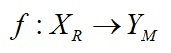Sep 29, 2023

channels

Education

# Introduction to Bijective Physics

Maya of 'God Particle' : Shiva Physics - I

For more than 100 years physics is building the models in which reality is squeezed in different unnatural forms. The result of this "forced" methodology is that we are now discovering things which do not exist. It is time to rebuild physics accordingly to the shapes of reality.

Bijective physics is using bijective research methodology in order to build “two-way” bridge between models of reality and reality itself. In Bijective physics every single element in a given model has the exactly one element in physical reality. A given element of the reality Xand a given correspondent element of the model YM  are related by bijective function:In today physics often happens that a given model is build on purely theoretical predictions without being observed directly by senses or indirectly by instruments. Such an example is the idea that all elementary particles are mass-less and that some field exists which gives mass to the particles. The idea was born as the result of Super-symmetry model SUSY which predicts that every elementary particle should have its “super-partner” particle. Higgs mechanism is mathematically correct, but it has no basis in human perception (direct perception or indirect perception by instruments). It is not in accord with the “mass-energy equivalence principle” and formula E = mc2 which clearly confirms that mass of the given elementary particle is equal the content of its energy, so mass has the origin in the energy of a given particle.

The idea that all particles are “mass-less” is false and has lead to the extremely complicated model of the Higgs mechanism, which cannot answer the most simple questions, as for example: “About 99% of proton mass are gluons. Higgs field does not interact with the gluons. How Higgs field can give mass to the proton”?

Bijective Physics offers a model where mass of elementary particles is elegantly described without the introduction of Higgs field.

Next example of the model which has no bijective correspondence with reality is the idea of “negative mass” in order to solve the enigma of dark energy and dark matter. Such mathematical proposals are highly complicated physics and do not bring any constructive solutions. The term “negative mass” is mathematically correct, but it has no meaning and should be not introduced in physics. It does not pass bijective analysis.

The same is valid for the model of “negative energy”. The idea was introduced by Hawking in his book The Brief History of Time, where he has presented energy of matter as “positive energy” and energy of gravity as “negative energy”; their sum in the universe is always zero: Em +( – Eg) = 0. Hawking suggests that in the “inflation period these two energies are getting multiplied. It is right that (1) + (-1) = 0. But this does not mean that energy can be produced in the universe on the basis of this equation.

A 100 years old model of cosmology and physics, namely, that space is “empty” is false idea. We cannot bring the model of Euclidean mathematical space in physics and believe universal space has only “geometrical properties”. Such a model has created the unsolvable problems in physics, which bijective physics is slowing elegantly.

Continued to "Higgs Mechanism Demolition"

02-Mar-2019

More by :  Amrit Sorli

Name *

Email ID

Comment *

Characters
Verification Code*